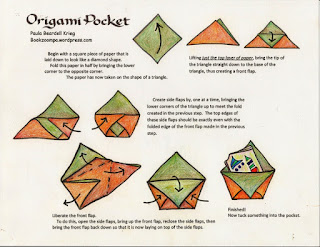### Measurements, Significant Figures, and Density

The first unit in chemistry class includes measurements and general number sense. There are several labs that we do and review as a class to get the idea of significant figures.  One of these labs involves determining the thickness of aluminum foil. First the students had a warm-up as the bell-ringer:

#### Class Warm-up:  Density

Remember the relationships:
Density = mass/Volume and  Volume = length x width x height.

These two equations can be combined to yield:
m
D = -----------------------------
l x w x h
If you wanted to know how “tall” something is, you would solve for h, as in the equation following:

m
h = -----------------------------
D x l  x w

Solve the following problems:1.       What is the volume of a block that is 8.20 cm long, 2.25 cm wide, and 1.00 cm high?
2.       If the density of a substance is 0.525 g/cm3, and the volume of a sample is 18.25 cm3, what is the mass of the sample?
3.       A piece of paper is known to have an area of 595.5 cm2 and a mass of 4.589 g. What is the thickness (height) of this paper, if paper has a density of 0.72 g/cm3?

#### Class Activity: Foiled

Pre-cut squares of three types of aluminum foil were set out, and students were directed to take the measurements needed to calculate the thickness, based on the warm-up calculation above. The density of aluminum was given to the students as 2.70 g/cm3. I tried to cut the squares so that they were not an exact centimeter size, so that students would need to read the mm lines and estimate a "between the lines" digit.Pre-cut squares of foil saves time. I made quick origami envelopes to store them in.Origami pocket directions